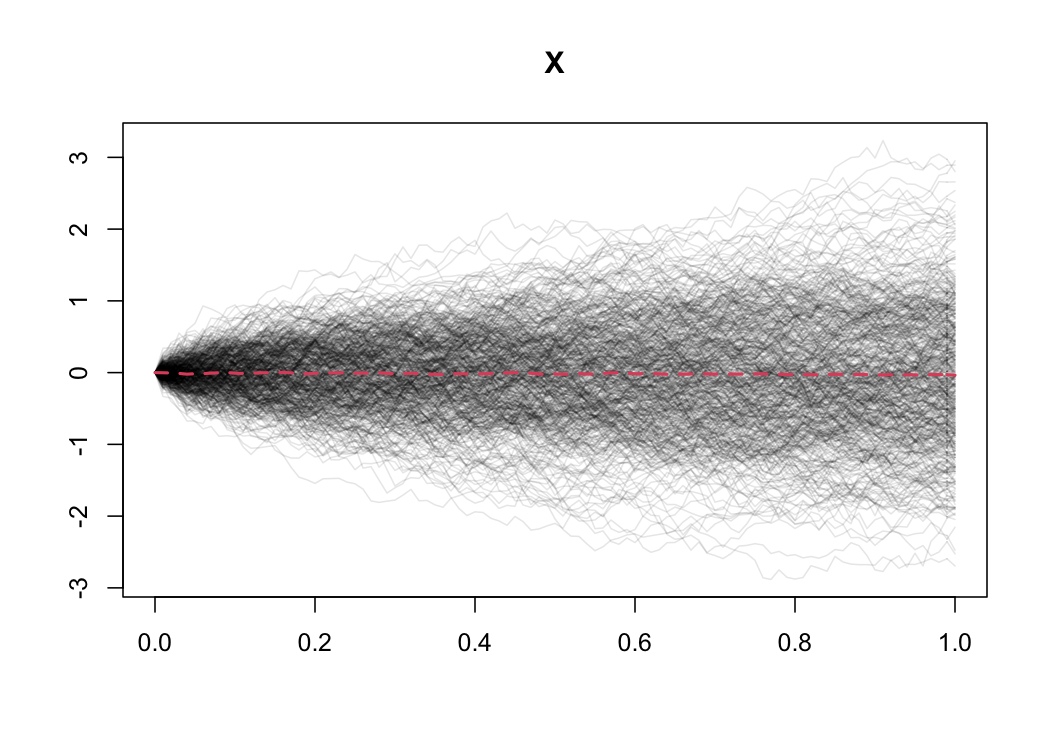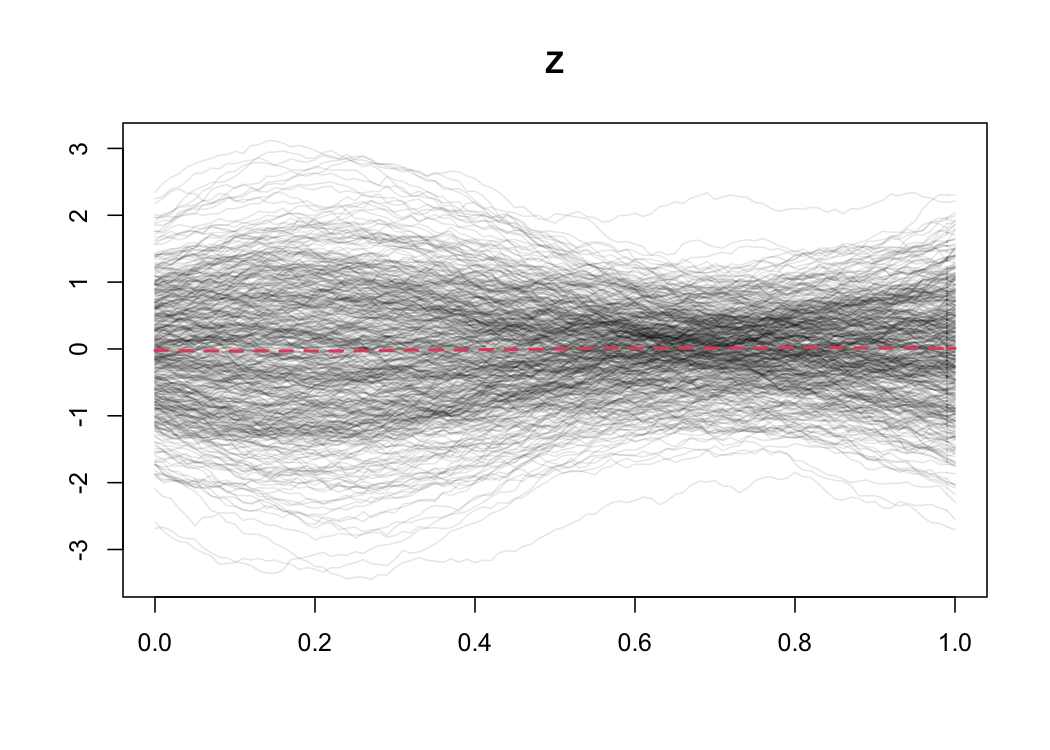# Introduction

ghcm is an R package used to perform conditional independence tests for densely observed functional data.

This vignette gives a brief overview of the usage of the ghcm package. We give a brief presentation of the idea behind the GHCM and the conditions under which the test is valid. Subsequently, we provide several examples of the usage of the ghcm package by analysing a simulated dataset.

# The Generalised Hilbertian Covariance Measure (GHCM)

In this section we briefly describe the idea behind the GHCM. For the full technical details and theoretical results, see .

Let $$X$$, $$Y$$ and $$Z$$ be random variables of which we are given $$n$$ i.i.d. observations $$(X_1, Y_1, Z_1), \dots, (X_n, Y_n, Z_n)$$ and where $$X$$, $$Y$$ and $$Z$$ can be either scalar or functional. Existing methods, such as the GCM  implemented in the GeneralisedCovarianceMeasure package , can deal with most cases where both $$X$$ and $$Y$$ are scalar hence our primary interest is in the cases where at least one of $$X$$ and $$Y$$ are functional. For the moment, we think of all functional observations as being fully observed.

The GHCM estimates the expected conditional covariance of $$X$$ and $$Y$$ given $$Z$$, $$\mathscr{K}$$, and rejects the hypothesis $$X \mbox{{}\perp\mkern-11mu\perp{}}Y \,|\,Z$$ if the Hilbert-Schmidt norm of $$\mathscr{K}$$ is large. To describe the algorithm, we utilise outer products $$x \otimes y$$, that can be thought of as a possibly infinite-dimensional generalisation of the matrix outer product $$xy^T$$ (for precise definitions, we refer to ).

1. Regress $$X$$ on $$Z$$ and $$Y$$ on $$Z$$ yielding residuals $$\hat{\varepsilon}$$ and $$\hat{\xi}$$, respectively.
2. Let $$\mathscr{R}_i = \hat{\varepsilon}_i \otimes \hat{\xi}_i$$ and compute the test statistic $T = \left\| \frac{1}{\sqrt{n}} \sum_{i=1}^n \mathscr{R}_i \right\|_{HS}.$
3. Estimate the covariance of the limiting distribution $\hat{\mathscr{C}} = \frac{1}{n-1} \sum_{i=1}^n (\mathscr{R}_i - \bar{\mathscr{R}}) \otimes_{HS} (\mathscr{R}_i - \bar{\mathscr{R}}),$ where $$\bar{\mathscr{R}} = n^{-1} \sum_{i=1}^n \mathscr{R}_i$$.
4. Sample independent $$W_1, \dots, W_B \sim \| \mathcal{N}(0, \hat{\mathscr{C}}) \|_{HS}$$ and produce a $$p$$-value by setting $p = \frac{1+\sum_{b=1}^B \mathbf{1}_{\{ W_b > T \} } }{B+1}.$

Assuming that the regression methods perform sufficiently well, the GHCM has uniformly distributed $$p$$-values when the null is true. It should be noted that there are situations where $$X \mbox{{}\not\!\perp\mkern-11mu\perp{}}Y \,|\,Z$$ but the GHCM is unable to detect this dependence for any sample size, since $$\mathscr{K}$$ can be zero in this case.

In practice, we do not observe the functional data fully but rather at a discrete set of values. To deal with this, we utilise functional principal components analysis (FPCA) to express $$\hat{\varepsilon}$$ and $$\hat{\xi}$$ (in the case that these are functional) as vectors.

# Example applications on a simulated dataset

To give concrete examples of the usage of the package, we perform conditional independence tests on a simulated dataset consisting of both functional and scalar variables. The functional variables are observed on a common equidistant grid of $$101$$ points on $$[0, 1]$$.

library(ghcm)
set.seed(111)
data(ghcm_sim_data)
grid <- seq(0, 1, length.out=101)
colnames(ghcm_sim_data)
#>  "Y_1" "Y_2" "X"   "Z"   "W"

ghcm_sim_data consists of 500 observations of the scalar variables $$Y_1$$ and $$Y_2$$ and the functional variables $$X$$, $$Z$$ and $$W$$. The curves and the mean curve for functional data can be seen in Figures 1, 2 and 3.Figure 1: Plot of $$X$$ with the estimated mean curve in red.Figure 2: Plot of $$Z$$ with the estimated mean curve in red.